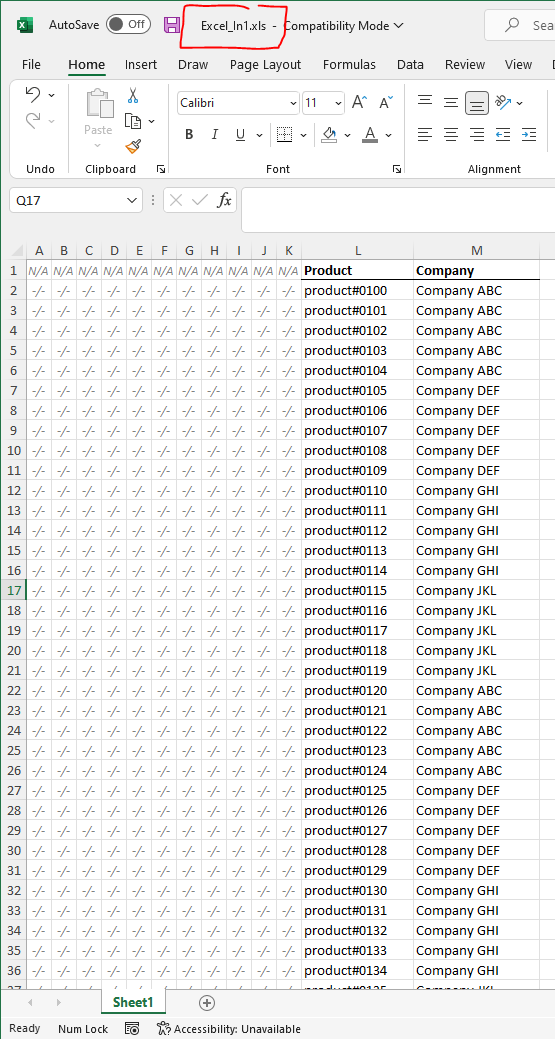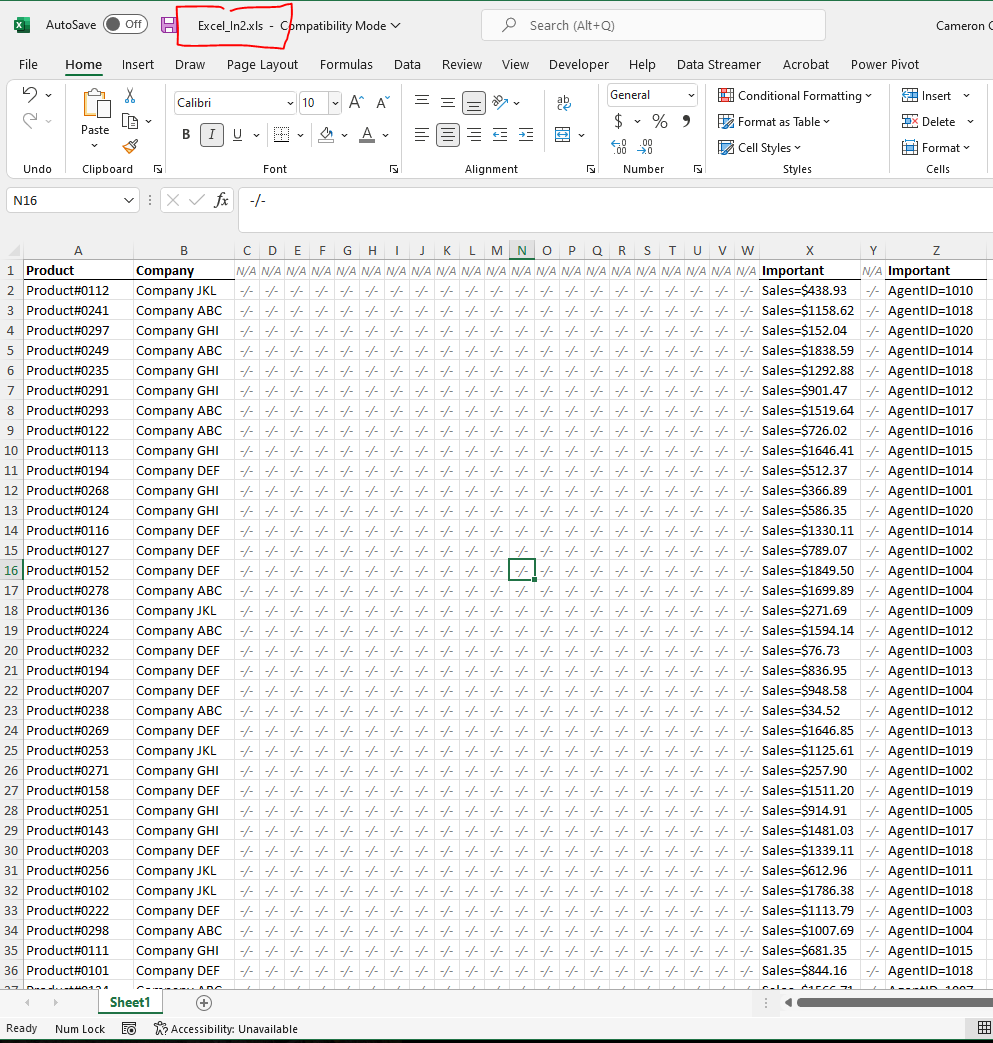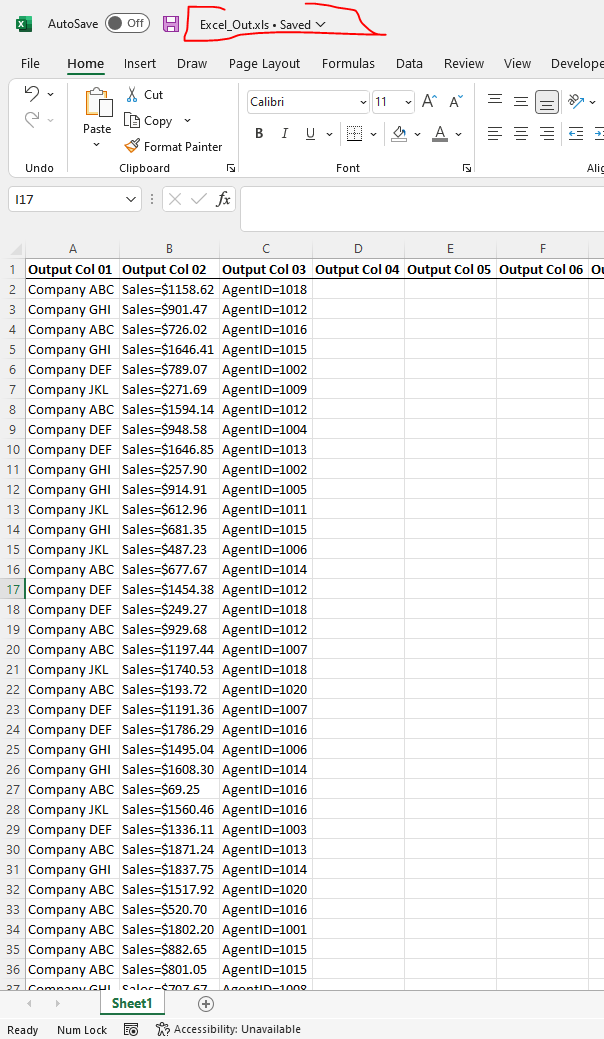# [Solved] Handling pairs of pattern matching in multiple excel files through VB macros

Your setup as best I could understand it:And… This is the code I wrote:

``````Option Explicit
Option Base 1
Sub CopyData()

Dim XLout As Workbook   'Excel_Out.xls
Dim XLin1 As Workbook   'Excel_In1.xls
Dim XLin2 As Workbook   'Excel_In2.xls
Dim ProductList         'Product/Company List from XLin1
Dim ProductListO()      'Concatenated Version of above
Dim DataList            'Product/Company List from XLin2
Dim DataXcol            'Extra Data to pull from Column X in XLin2
Dim DataZcol            'Extra Data to pull from Column Z in XLin2
Dim Output()            'Output Array for XLout
Dim i As Long           'Iterations
Dim counter As Long     'Item number
Dim TimeCount

TimeCount = Timer

' >>> All Workbooks
Set XLout = ThisWorkbook
Set XLin1 = Workbooks.Open("C:\Users\ccritchlow\Documents\A\Test\Excel_In1.xls")
Set XLin2 = Workbooks.Open("C:\Users\ccritchlow\Documents\A\Test\Excel_In2.xls")

' >>> Store Source Data in Arrays
With XLin2.Sheets(1)
DataList = .Range("A2:B" & .Range("A" & Rows.Count).End(xlUp).Row)
DataXcol = .Range("X2:X" & .Range("A" & Rows.Count).End(xlUp).Row)
DataZcol = .Range("Z2:Z" & .Range("A" & Rows.Count).End(xlUp).Row)
End With

' >>> Store Product List Data in Arrays
ProductList = XLin1.Sheets(1).Range("L2:M" & XLin1.Sheets(1).Range("M" & Rows.Count).End(xlUp).Row)
ReDim ProductListO(1 To UBound(ProductList, 1))
For i = 1 To UBound(ProductList, 1)
ProductListO(i) = ProductList(i, 1) & "-" & ProductList(i, 2)
Next i

' >>> Move entries from XLin2 (that exist on XLin1) into "Output" Array
ReDim Preserve Output(UBound(DataList, 1), 3)
counter = 1
For i = 1 To UBound(DataList, 1)
DataList(i, 1) = DataList(i, 1) & "-" & DataList(i, 2)
If Not IsError(Application.Match(DataList(i, 1), ProductListO(), 0)) Then
Debug.Print
Output(counter, 1) = DataList(i, 2)
Output(counter, 2) = DataXcol(i, 1)
Output(counter, 3) = DataZcol(i, 1)
counter = counter + 1
End If
Next i

' >>> Output to XLout
XLout.Sheets(1).Range("A2").Resize(UBound(Output, 1), 3) = Output()

Application.StatusBar = "Total Time to review " & UBound(DataList, 1) & " lines = " & Timer - TimeCount

End Sub
``````

It does the following

• Is stored on “Excel_Out.xls”
• Opens both “Excel_In#.xls” workbooks
• Stores all required data in arrays
• Identifies data on XLin2 whose “company&productname” exist on XLin1
• Outputs that data to “Excel_Out.xls”

This is how it looks:solved Handling pairs of pattern matching in multiple excel files through VB macros